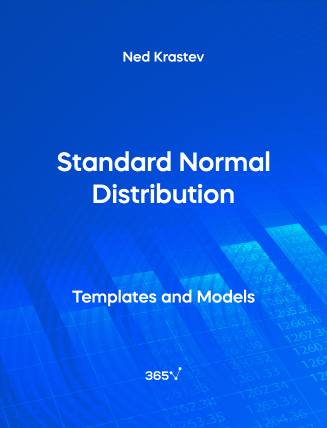## Standard Normal Distribution – Excel Template###### Ned Krastev

The normal distribution is the most widely used type of distribution in the world of finance. A number of models, statistical tools, and pricing methods rely on it. The standard normal distribution is a particular type of normal distribution that has a mean of 0 and a standard deviation of 1.

The normal distribution is bell-shaped and absolutely symmetrical. The mean, median, and mode of the normal distribution coincide. And it is fully described by two parameters—mean and variance. For this reason, we can indicate the probability of observing a given value when using a normal distribution and express that as a distance from the mean.

This open-access Excel template is a practical tool for statisticians, business intelligence analysts, data scientists, portfolio managers, risk managers, and anyone studying finance. Subscribe to our extensive Statistics course to discover how essential measures apply in the real world.

Other open-access templates you may want to explore include—Measures of Central Tendency, Uniform Distribution, and Binomial Distribution.Top